Basics of Derivative Pricing and Valuation | IFT World
IFT Notes for Level I CFA® Program
IFT Notes for Level I CFA® Program

# Part 1

## 1.  Introduction

• How does the pricing of the underlying asset affect the pricing of derivatives?
• How are derivatives priced using the principle of arbitrage?
• How are the prices and values of forward contracts determined?
• How are futures contracts priced differently from forward contracts?
• How are the prices and values of swaps determined?
• How are the prices and values of European options determined?
• How does American option pricing differ from European option pricing?

## 2. Basic Derivative Concepts, Pricing the Underlying

### 2.1.     Basic Derivative Concepts

This is a recap of what we saw in the previous reading.

Forward contract: It is a customized over-the-counter derivative contract in which two parties agree that one party, the buyer, will purchase an underlying asset from the other party, the seller, at a later date, at a fixed price they agree upon when the contract is signed.

Futures contract: It is a standardized derivative contract created and traded on a futures exchange in which two parties agree that one party, the buyer, will purchase an underlying asset from the other party, the seller, at a later date at a price agreed upon by the two parties when the contract is initiated. There is also a daily settling of gains and losses and a credit guarantee by the futures exchange through its clearinghouse.

Swap contract: It is an over-the counter derivate contract in which two parties agree to exchange a series of cash flows whereby one party pays a variable series that will be determined by an underlying asset or rate, and the other party pays either 1) a variable series determined by a different underlying asset or rate or 2) a fixed series.

Option contract: It is a derivative contract in which one party, the buyer, pays a sum of money to the other party, the seller or writer, and receives the right to buy or sell an underlying asset at a fixed price either on a specific expiration date or at any time prior to the expiration date.

### 2.2.     Pricing the Underlying

The price or value of a financial asset is the expected future price plus any benefits such as dividends or coupon interest minus costs discounted at a rate appropriate for the risk assumed. The exhibit below shows how to get the current price  of an asset by discounting its expected future price , by the risk-free rate r, plus the risk premium , over the period from 0 to T.

Source: CFA Program Curriculum, Basics of Derivative Pricing and Valuation

The price of the underlying at time t = 0 is given by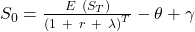Let us now decompose this expression and understand what each of the terms mean.

• Expected future valueE(ST): Let’s say that the expected future value of the underlying asset a year later at t = 1 is 100. It cannot be known for sure and its value a year later is given by the normal distribution, E(ST) is the expected value.
• Discount rate: The first term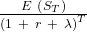is the present value of expected future price of an asset with no interim cash flows (in our case, 100) where r = risk-free rate. Given that the value in the future is uncertain, it is not appropriate to discount it at the risk-free rate, so a risk premium λ is added to the risk-free rate. If the riskiness of the asset is high, then λ is high.
• Present value of the asset is then given by•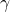is the present value of any benefits of holding the asset between t = 0 and t = 1.is added to the current price. It is assumed that the benefits are certain, so their future value is discounted at the risk-free rate to calculate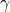. includes both monetary and non-monetary benefits. For an asset like stocks,dividend paid is an example of monetary benefit. Convenience yield is the non-monetary benefit of holding an asset. The pleasure one derives from looking at one’s art collection or gold is an example of non-monetary benefit.
•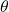is the present value of the costs of holding an asset between t = 0 and t = 1. It is subtracted from the current price. For example, if the asset is gold and you rent a locker to store it safely, then this cost incurred that must be subtracted from the current price.
• Cost of carry is the net cost of holding an asset. Cost of carry =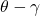## 3. The Principle of Arbitrage

Arbitrage is a type of transaction undertaken when two assets or portfolios produce identical results but sell for different prices. Let us take the example below:

Source: CFA Program Curriculum, Basics of Derivative Pricing and Valuation

Interpretation:

• The exhibit above is self-explanatory. Assets A and B have the same values at time T, but initially at time t = 0, asset A sells for lesser than asset B. This leads to an arbitrage opportunity.
• For example, at t = 0, assume A is priced at 100 and B is priced at 101. If you borrow B and sell it, and buy A for 100, the net cash is 101 – 100 = 1.
• At time t = T, both A and B are priced the same. Sell asset A. Use the proceeds to buy B and return it since it was borrowed initially. The net cash flow is 0.

Arbitrage opportunities are exploited quickly and hence do not last for long. In the above example, when the arbitrage opportunity exists, the demand for A will go up causing its price to go up. Similarly, when more people sell B, its demand goes down, leading to a decrease in its price causing the prices of both the assets to come to the same level. It is not just the difference in price that matters; one needs to consider the transaction costs as well. If the transaction costs exceed the benefit from an arbitrage opportunity, then it is not worth exploiting.

Law of one price: If two assets have the same expected return in the future, then their market price today must be the same.

Arbitrage and Derivatives

The price of a derivative is tied to the price of the underlying. For example, if the price of a stock goes up, then a call option on the stock also goes up. Therefore, a derivative can be used to hedge an underlying, or vice versa. For example, if you are long on Google stock, then you can either short the call option or buy a put option to eliminate your risk.

Since the risk is eliminated, the expected return is the risk-free return.

A derivative must be priced such that no arbitrage opportunities exist, and there can be only one price for the derivative that earns the risk-free return. If it earns a return in excess of the risk-free rate, then arbitrage opportunities exist for (underlying + derivative) position.

Arbitrage and Replication

An asset and a derivative can be combined to produce a risk-free bond in one of the ways shown below. Conversely, an asset and the risk free asset can be combined to produce a derivative.

There are two ways to replicate when the underlying asset is a stock:

• Buy stock + put option = risk-free rate
• Buy stock – risk-free rate = short put option

Replication is the creation of an asset or portfolio from another asset, portfolio, and/or derivative. Why is replication needed? Isn’t it easier to just buy a government security to earn the risk-free rate instead of buying the asset and a derivative? There are some situations under which replication is valuable:

• When transaction costs are lower.
• When one of the components, say the derivative, is not rightly priced resulting in an arbitrage opportunity.

Risk Aversion, Risk Neutrality, and Arbitrage-Free Pricing

Risk aversion: Most investors are risk-averse and expect a compensation (risk premium) for assuming risk. As we have seen earlier, a derivative can be combined with an asset to mitigate the risk and produce a risk-free asset. We saw in the previous section what factors affect a derivative’s pricing. Since risk aversion of an investor does not impact derivative pricing, one can derive the derivative price assuming investors are risk-neutral. When pricing derivatives, use the risk-free rate, i.e. the price of a derivative can be calculated by discounting it at the risk-free rate rather than the risk-free rate plus a risk premium.

When pricing assets, a risk premium is added to the risk-free rate. Recall the first term of current price Sof an asset iswhere λ is the risk premium. This means that the asset’s price in the spot market factors in the risk aversion of an investor. Since the risk aversion is already captured in the asset pricing, it is not included in a derivative’s pricing.

Risk-neutral pricing: Derivatives pricing is sometimes called risk-neutral pricing because there is only one derivative price, which combined with the underlying asset, can earn the risk-free rate.

Arbitrage-free pricing: The overall process of pricing derivatives by arbitrage and risk-neutrality is called arbitrage-free pricing. It is also called the principle of no arbitrage. Limitations to execute arbitrage transactions include:

• It may not be possible to short sell assets.
• Information on arbitrage opportunities/volatility of the asset may not be easily accessible, and it can be risky to execute if one lacks accurate information on the inputs.
• Arbitrage requires capital which may not be easily available to everyone to maintain positions. For instance, if exploiting an arbitrage opportunity requires \$100 million, it is not easy to borrow so much money to engage in a transaction.

Clearinghouses do not place any restrictions on transactions that can be arbitraged.

hedge portfolio is one that eliminates arbitrage opportunities and implies a unique price for a derivative.

## 4. Pricing and Valuation of Forward Contracts: Pricing vs. Valuation; Expiration; Initiation

This section lays the foundation for the subsequent sections. We look at two terms, price and value, and what they mean in context to different assets/derivatives.

Stock price vs. value

• The price of a stock is its market price while the value of a stock is its intrinsic value often determined by fundamental analysis (by analyzing a company’s financial statements, and discounting future cash flows). Alternatively, the book value of a company is compared to its market value.
• For instance, if the intrinsic value of a stock is 110 and its market price is 100, then it means investors must buy the stock.

Option contract price versus value

• The price is similar to what we saw above for a stock. For instance, the price of a call option is its market price or what you would pay to buy the call option. The value of a call option is based on the underlying and other variables that impact the option.

Forwards, futures, and swaps

• Unlike stocks, bonds, or options, these do not require an initial cash outlay.
• Their price and value cannot be compared to each other.
• The price of a forward contract is the fixed price or rate that is embedded in the contract; it represents the price or rate at which the underlying will be purchased at a later date.
• The value changes over the life of the contract. It is zero at initiation. Over time, as spot price or rate changes, the value to each party changes. If the price of the underlying increases, then the value to the long also increases.